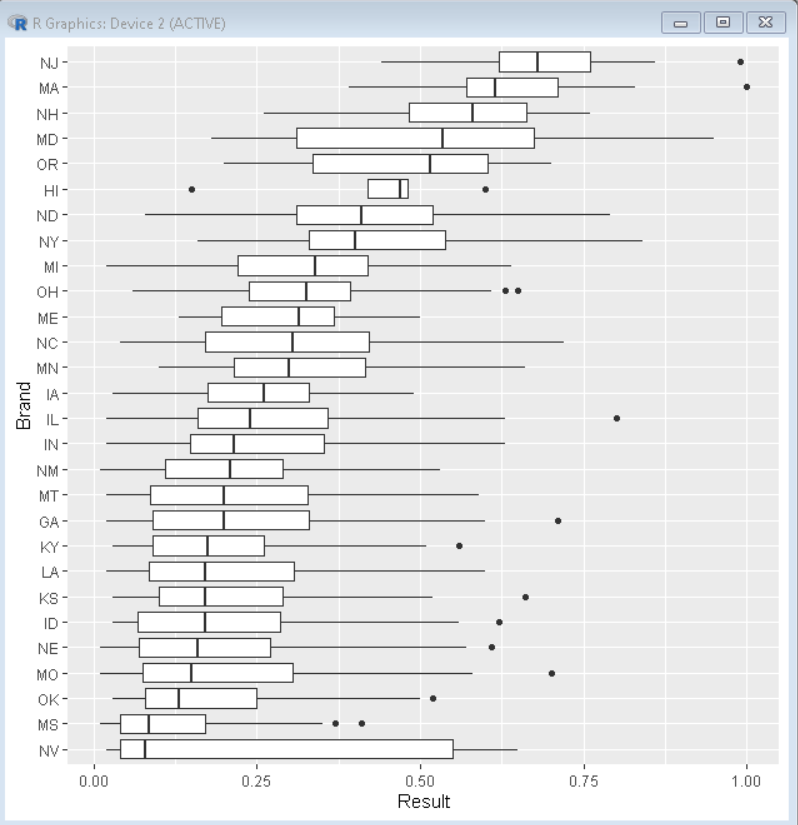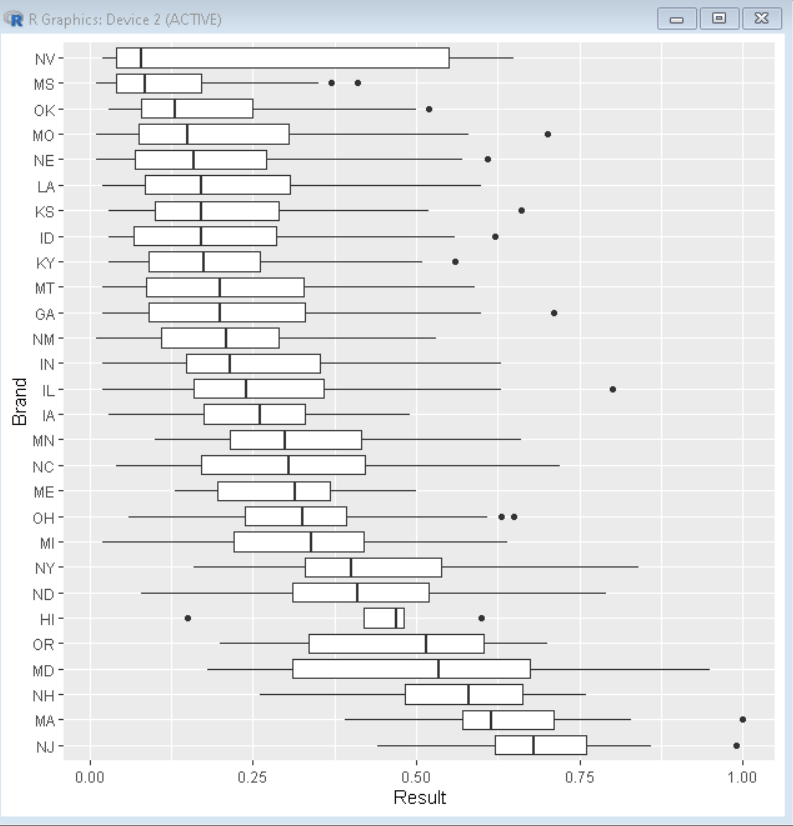GFG App
Open AppBrowser
Continue

# How to order boxes in boxplot with fct_reorder in R?

In this article, we will discuss how to reorder boxes in boxplot with the fct_reorder() function in the R Programming Language.

By default, The ggplot2 boxplot orders the boxes in alphabetical order of categorical variable. But for better visualization of data sometimes we need to reorder them in sorted order. To sort the data in ascending or descending order, we use the fct_reorder() function of the forcats package. The forcats package of the R Language contains helpers for reordering and modifying factor levels. The fct_reorder() function helps us to reorder factor levels by sorting along with another variable.

## Method 1: Reorder boxplot in ascending order

The fct_reorder() function by default sorts the data in the ascending order of value_variable. So, we use the fact_reorder() function to sort the data first in ascending order. Then we use the geom_boxplot() function of the ggplot2 package to plot the boxplot.

Syntax: df %>% mutate( categorical_variable= fct_reorder( categorical_variable, value_variable))

Parameters:

• df: determines the data frame to be used for reordering of data.
• categorical_variable: determines the variable which is to be reordered.
• value_variable: determines the variable according to which data is to be reordered.

Syntax to install and import tidyverse package:

```install.package('tidyverse')    # To install
library(tidyverse)              # To import  ```

Example:

Here, is a basic boxplot with boxes sorted in ascending order. The CSV used in the example can be downloaded here.

## R

 `# load library tidyverse ` `library``(tidyverse) ` ` `  `# load sample data ` `sample_data <- ``read.csv``(``"sample_box.CSV"``) ` ` `  `# Reorder data with fct_reorder function  ` `# and plot boxplot ` `sample_data <- sample_data%>%``mutate``(Brand=``fct_reorder``(Brand, Result)) ` ` `  `# plot boxplot ` `ggplot``(sample_data, ``aes``(x=Result, y=Brand))+ ` `          ``geom_boxplot``()`

Output:## Method 2: Reorder boxplot in descending order

To reorder data in descending order, we use the .desc argument of the fct_reorder() function. The .desc argument when true, sorts the data in descending order, by default it is false thus giving data in ascending order.

Syntax: df %>% mutate( categorical_variable= fct_reorder( categorical_variable, value_variable, .desc))

Parameters:

• .desc: determines a boolean value, which if true sorts the data in descending order. By default, it is false.

Example:

Here, is a basic boxplot with boxes sorted in descending order.

## R

 `# load library tidyverse ` `library``(tidyverse) ` ` `  `# load sample data ` `sample_data <- ``read.csv``(``"sample_box.CSV"``) ` ` `  `# Reorder data with fct_reorder function  ` `# and plot boxplot ` `sample_data <- sample_data%>%``mutate``(Brand=``fct_reorder``( ` `  ``Brand, Result, .desc=``TRUE``)) ` ` `  `# plot boxplot ` `ggplot``(sample_data, ``aes``(x=Result, y=Brand))+ ` `          ``geom_boxplot``()`

Output:My Personal Notes arrow_drop_up